## RS Aggarwal Class 6 Solutions Chapter 10 Ratio, Proportion and Unitary Method Ex 10A

These Solutions are part of RS Aggarwal Solutions Class 6. Here we have given RS Aggarwal Solutions Class 6 Chapter 10 Ratio, Proportion and Unitary Method Ex 10A.

Other Exercises

Question 1.
Solution:
(i) 24 to 56
= $$\\ \frac { 24 }{ 56 }$$
= $$\frac { 24\div 8 }{ 56\div 8 }$$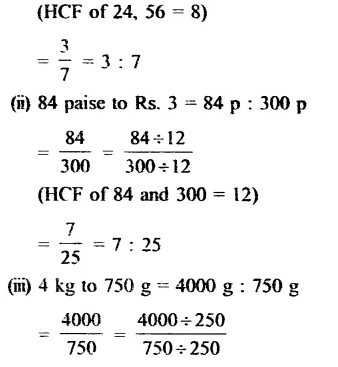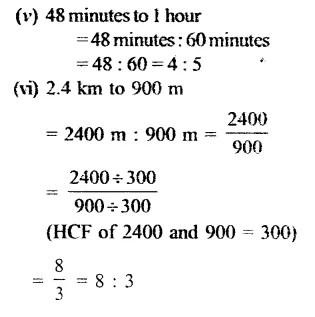Question 2.
Solution:
(i) 36 : 90
= $$\\ \frac { 36 }{ 90 }$$
= $$\frac { 36\div 18 }{ 90\div 18 }$$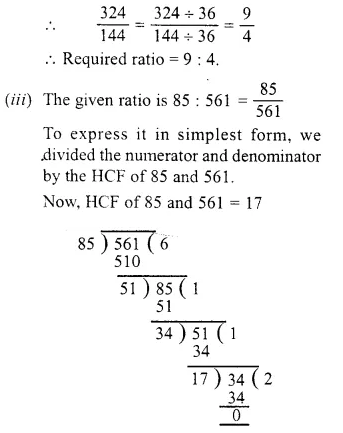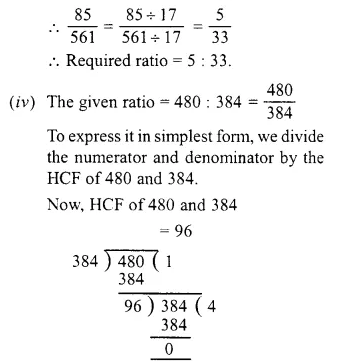Question 3.
Solution:
(i) The given ratio = Rs. 6.30 : Rs. 16.80
= $$\\ \frac { Rs.6.30 }{ Rs.16.80 }$$
= $$\\ \frac { 630 }{ 1680 }$$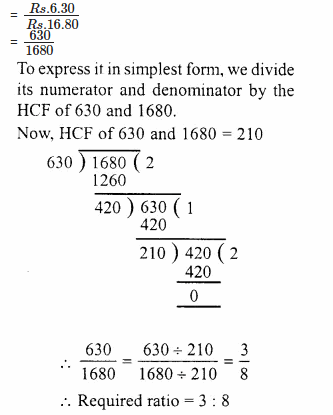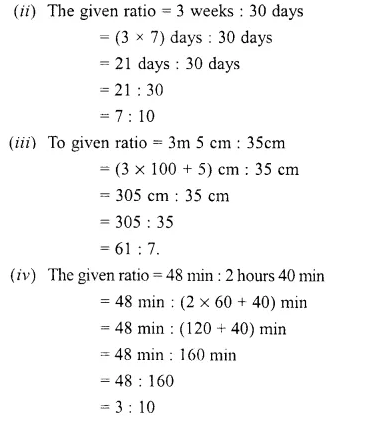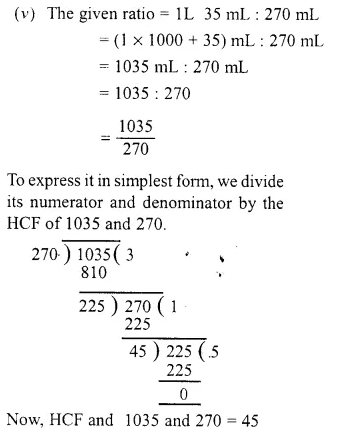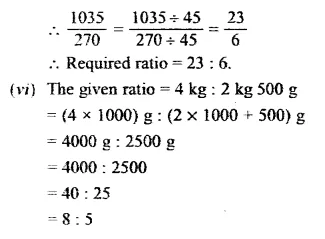Question 4.
Solution:
Earning of Sahai = Rs. 16800
and of his wife = Rs. 10500
Then total income = Rs. 16800 + 10500
= Rs. 27300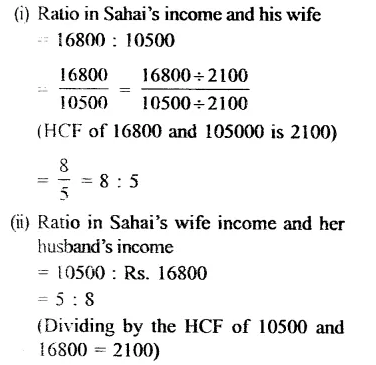Question 5.
Solution:
Rohit monthly earnings = Rs. 15300
and his savings = Rs. 1224
So, his expenditure = Rs. 15300 – 1224
= Rs. 14076
Now,Question 6.
Solution:
Let the number of male and female workers in the mill be 5x and 3x respectively. Then,
5x = 115
=> $$\\ \frac { 5x }{ 5 }$$ = $$\\ \frac { 115 }{ 5 }$$
(Dividing both sides by 5)
=> x = 23
Number of female workers in the mill
= 3x
= 3 x 23 = 69.

Question 7.
Solution:
Let the number of boys and girls in the school be 9x and 5x respectively.
According to the question,
9x + 5x = 44
=> 14x = 448
=> $$\\ \frac { 14x }{ 14 }$$ = $$\\ \frac { 448 }{ 14 }$$
(Dividing both sides by 14)
=> x = 32.
Number of girls =5x
= 5 x 32
= 160

Question 8.
Solution:
Total amount = Rs. 1575
Ratio in Kamal and Madhu’s share = 7 : 2
Sum of ratios = 7 + 2 = 9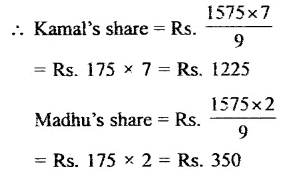Question 9.
Solution:
Total amount = Rs. 3450
Ratio in A, B and C shares = 3 : 5 : 7
Sum of share = 3 + 5 + 7 = 15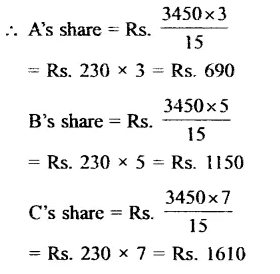Question 10.
Solution:
Let the numbers be 11x and 12x.
Then. 11x + 12x = 460
=> 23x = 460Question 11.
Solution:
Length of line segment = 35 cm
Ratio = 4 : 3
Sum of ratio = 4 + 3 = 7Question 12.
Solution:
Total bulbs produced per day = 630
Out of every 10 bulbs, defective bulb = 1
Out of every 10 bulbs, lighting bulbs = 10 – 1 = 9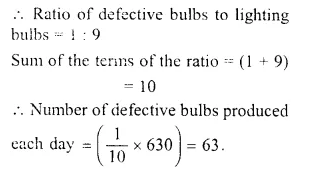Question 13.
Solution:
Price of 20 pencils = Rs. 96
(1 score = 20 pencils)
Price of 1 pencil = Rs. (96 ÷ 20)
= Rs. 4.80
Price of 12 ball pens = Rs. 50.40
(1 dozen = 12)
Price of 1 ball pen = Rs. (50.40 ÷ 12)
= Rs. 4.20.
Ratio of the price of a pencil to that of a ball pen = Rs. 4.80 : Rs. 4.20
= 480 paise : 420 paise
= 480 : 420
= 48 : 42
= 8 : 7.
Required ratio = 8 : 7.

Question 14.
Solution:
It is given that the ratio of the length of a field to its width is 5 : 3.
If the width of the field is 3 metres then length = 5 metres.
If the width of the field is 1 metres than length = $$\\ \frac { 5 }{ 3 }$$ metres.
If the width of the field is 42 metres then length
= $$\\ \frac { 5 }{ 3 }$$ x 42 metres
= 5 x 14 metres
= 70 metres.

Question 15.
Solution:
Ratio in income and savings of a family = 11 : 2
But Total savings = Rs. 1520
Let income = x
11 : 2 = x : 1520
=> x = $$\\ \frac { 11\times 1520 }{ 2 }$$ = 11 x 760
= Rs 8360
Expenditure = total income – savings
= Rs 8360 – 1520
= Rs 6840

Question 16.
Solution:
Ratio in income and expenditure = 7 : 6
Total income = Rs. 14000
Let expenditure = x, then
7 : 6 :: 14000 : x
=>x = $$\\ \frac { 6\times 14000 }{ 7 }$$ = Rs. 12000
Savings = Total income – Expenditure
= Rs. 14000 – 12000
= Rs. 2000

Question 17.
Solution:
It is given that the ratio of zinc and copper in an alloy is 7 : 9.
If the weight of zinc in the alloy is 7 kg then the weight of copper in the alloy is 9 kg.Question 18.
Solution:
A bus covers in 2 hours = 128 km
128 It will cover in 1 hour = $$\\ \frac { 128 }{ 2 }$$ = 64 km
A train cover in 3 hours = 240 km
It will cover in 1 hour = $$\\ \frac { 240 }{ 3 }$$
= 80 km
Ratio in their speeds = 64: 80
= 4 : 5
{Dividing by 16, the LCM of 64, 80}

Question 19.
Solution:
(i) (3 : 4) or (9 : 16)
LCM of 4, 16 = 16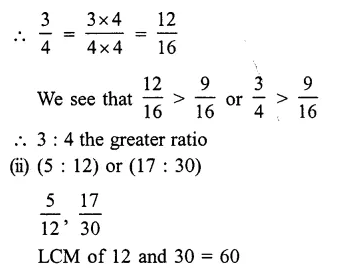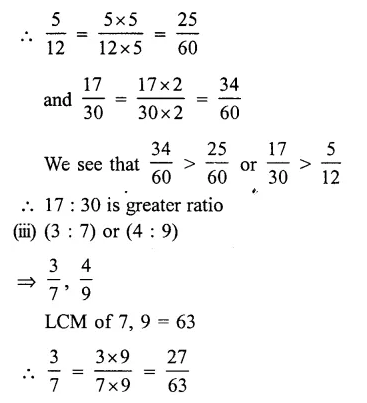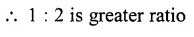Question 20.
Solution: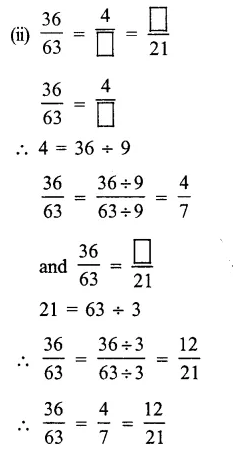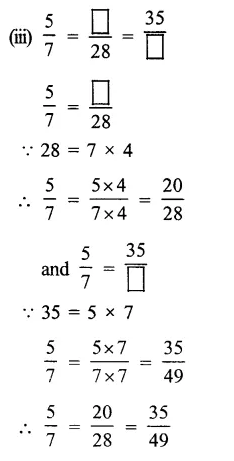Hope given RS Aggarwal Solutions Class 6 Chapter 10 Ratio, Proportion and Unitary Method Ex 10A are helpful to complete your math homework.

If you have any doubts, please comment below. Learn Insta try to provide online math tutoring for you.